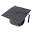IMIS | Vlaams Instituut voor de Zee

Vlaams Instituut voor de Zee

Platform voor marien onderzoek

# IMIS

Publicaties | Instituten | Personen | Datasets | Projecten | Kaarten
 [ meld een fout in dit record ] mandje (0): toevoegen | toonThe influence of porosity gradients on mixing coefficients in sedimentsMeysman, F.J.R.; Malyuga, V.S.; Boudreau, B.P.; Middelburg, J.J. (2007). The influence of porosity gradients on mixing coefficients in sediments. Geochim. Cosmochim. Acta 71(4): 961-973 In: Geochimica et Cosmochimica Acta. Elsevier: Oxford,New York etc.. ISSN 0016-7037; e-ISSN 1872-9533, meerBeschikbaar in Auteurs VLIZ: Open Repository 138655 [ OMA ]

 Trefwoord Marien/Kust

 Auteurs Top Meysman, F.J.R., meer Malyuga, V.S. Boudreau, B.P. Middelburg, J.J., meer

 Abstract The surface layer of aquatic sediments is a zone characterized by both porosity gradients and intensive mixing. In the standard approach, porosity gradients are ignored when estimating mixing intensity. Here, model formulations with both constant and varying porosity are contrasted to estimate mixing coefficients Db from tracer depth profiles. Complementing the well-known exponential solution of the constant-porosity model, we present a general solution to the variable-porosity model in terms of hypergeometric functions. When using these models in a forward way, the tracer activities predicted by the variable-porosity model are higher than those generated by the constant-porosity model. Similarly, when inverse modelling, Db values estimated by the variable-porosity model are systematically higher than those derived from the constant-porosity model. Still, differences in Db values remain relatively small. When applying both mixing models to excess 210Pb data profiles from slope sediments, a maximal difference of 30% is obtained between Db values, the average deviation being 16%. A systematic exploration of parameter space predicts a maximal underestimation of 60% when deriving Db values from the constant-porosity mixing model. Given the uncertainty imposed by other model assumptions underlying the diffusive mixing model, the influence of porosity gradients on Db values must be classified as rather modest. Hence, the current mixing coefficient database is not biased by the constant porosity approximation.

 Alle informatie in het Integrated Marine Information System (IMIS) valt onder het VLIZ Privacy beleid Top | Auteurs